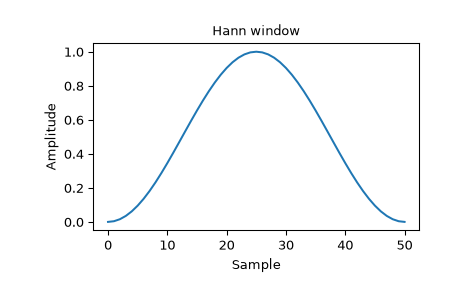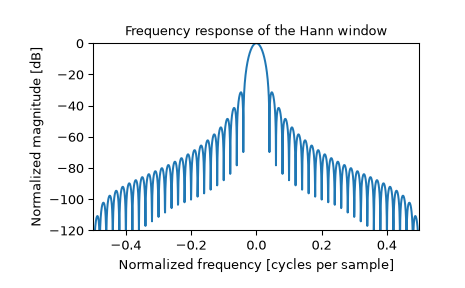# scipy.signal.windows.hann¶

scipy.signal.windows.hann(M, sym=True)[source]

Return a Hann window.

The Hann window is a taper formed by using a raised cosine or sine-squared with ends that touch zero.

Parameters
Mint

Number of points in the output window. If zero or less, an empty array is returned.

symbool, optional

When True (default), generates a symmetric window, for use in filter design. When False, generates a periodic window, for use in spectral analysis.

Returns
wndarray

The window, with the maximum value normalized to 1 (though the value 1 does not appear if M is even and sym is True).

Notes

The Hann window is defined as

$w(n) = 0.5 - 0.5 \cos\left(\frac{2\pi{n}}{M-1}\right) \qquad 0 \leq n \leq M-1$

The window was named for Julius von Hann, an Austrian meteorologist. It is also known as the Cosine Bell. It is sometimes erroneously referred to as the “Hanning” window, from the use of “hann” as a verb in the original paper and confusion with the very similar Hamming window.

Most references to the Hann window come from the signal processing literature, where it is used as one of many windowing functions for smoothing values. It is also known as an apodization (which means “removing the foot”, i.e. smoothing discontinuities at the beginning and end of the sampled signal) or tapering function.

References

1

Blackman, R.B. and Tukey, J.W., (1958) The measurement of power spectra, Dover Publications, New York.

2

E.R. Kanasewich, “Time Sequence Analysis in Geophysics”, The University of Alberta Press, 1975, pp. 106-108.

3

Wikipedia, “Window function”, https://en.wikipedia.org/wiki/Window_function

4

W.H. Press, B.P. Flannery, S.A. Teukolsky, and W.T. Vetterling, “Numerical Recipes”, Cambridge University Press, 1986, page 425.

Examples

Plot the window and its frequency response:

>>> from scipy import signal
>>> from scipy.fft import fft, fftshift
>>> import matplotlib.pyplot as plt

>>> window = signal.hann(51)
>>> plt.plot(window)
>>> plt.title("Hann window")
>>> plt.ylabel("Amplitude")
>>> plt.xlabel("Sample")

>>> plt.figure()
>>> A = fft(window, 2048) / (len(window)/2.0)
>>> freq = np.linspace(-0.5, 0.5, len(A))
>>> response = np.abs(fftshift(A / abs(A).max()))
>>> response = 20 * np.log10(np.maximum(response, 1e-10))
>>> plt.plot(freq, response)
>>> plt.axis([-0.5, 0.5, -120, 0])
>>> plt.title("Frequency response of the Hann window")
>>> plt.ylabel("Normalized magnitude [dB]")
>>> plt.xlabel("Normalized frequency [cycles per sample]")